# Basic Spatial Point Pattern Analysis in R

A wide variety of different phenomena can be modeled as points. These include physically static features like building locations, or dynamic events like moving vehicles or transient activities.

There are a variety of techniques for analyzing point data that can not only provide useful visualizations, but also provide useful insights that can inform action and programs. This tutorial will cover some of those techniques.

## Crime Data

Crime data is commonly made available as point data, with the points representing the location of a criminal act or an associated arrest. Since cities often make some subset of their crime data freely available on the internet, and many people find crime to be interesting, crime data is also useful for pedagogy.

Crime tends to cluster into hot-spots of repeated activity, and there are a number of theories that attempt to explain this spatial phenomena. On a practical level, identification of hot spots allows efficient targeting of both conventional enforcement resources, as well as interventions like problem-oriented policing that can address root causes of crime. While the long-term effects of targeting hot-spots are mixed, and can include simply displacing criminal activities to other areas, hot-spot analysis remains a useful law enforcement tool.

Police departments in most major cities make crime information available to the public on their websites. Some major cities make crime location data available as shapefiles or CSV files that can be imported into GIS for analysis. However, data is often presented on web maps contracted to vendors (such as LexisNexis or crimemapping.com) and cannot be conveniently downloaded. This seems especially prevalent with smaller cities and cities in politically-conservative areas of the country.

## The Modifiable Areal Unit Problem (MAUP)

One common technique for analyzing many point phenomena such as crime, is to aggregate (count the number of) events that occur within predefined areas like neighborhoods, zip codes, or census tracts.

However, the choice of what area to aggregate in can result in dramatically different analysis. If you modify the boundaries of aggregation areas, that affects which areas points are aggregated into and, therefore, the counts of points for each area. Boundaries of areas used for aggregation like neighborhoods or census tracts are often historical and political, and may not be perfectly suited to the aggregation analysis you are performing. This is the modifiable areal unit problem.

There is no universally applicable solution to the MAUP. In cases where accuracy is not essential (such as with data exploration) or possible (such as with data that has been aggregated for privacy reasons), the the uncertainty presented by the MAUP may be acceptable as long as caveats are provided along with the analysis. In other cases, more-sophisticated point-pattern analysis techniques like nearest-neighbor, kernel-density, or hot-spot analysis might be more appropriate.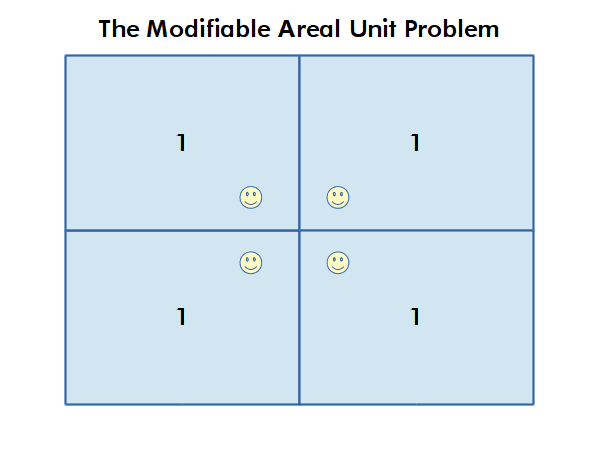The Modifiable Areal Unit Problem

## Exploring Crime Data

This example crime analysis will use crime data from the City of Denver Open Data Catalog. While most cities only make their data available on web maps, Denver is one of a handful of cities that publish unaggregated, georeferenced crime data in downloadable formats.

Loading the data with read.csv(), exploring the column names, and listing the first row gives some sense of what is available. The as.is=T parameter is needed so strings are not converted to factors, which makes them difficult to compare between different tables or objects.

```> data = read.csv('crime.csv', as.is=T)
>
> names(data)
 "INCIDENT_ID"            "OFFENSE_ID"             "OFFENSE_CODE"
 "OFFENSE_CODE_EXTENSION" "OFFENSE_TYPE_ID"        "OFFENSE_CATEGORY_ID"
 "FIRST_OCCURRENCE_DATE"  "LAST_OCCURRENCE_DATE"   "REPORTED_DATE"
 "INCIDENT_ADDRESS"       "GEO_X"                  "GEO_Y"
 "GEO_LON"                "GEO_LAT"                "DISTRICT_ID"
 "PRECINCT_ID"            "NEIGHBORHOOD_ID"        "IS_CRIME"
 "IS_TRAFFIC"
>
> crime[1,]
coordinates INCIDENT_ID   OFFENSE_ID OFFENSE_CODE
1229 (-105.0247, 39.70854)  2015734825 2.015735e+15         2404
OFFENSE_CODE_EXTENSION        OFFENSE_TYPE_ID OFFENSE_CATEGORY_ID
1229                      0 theft-of-motor-vehicle          auto-theft
FIRST_OCCURRENCE_DATE LAST_OCCURRENCE_DATE       REPORTED_DATE
1229   2015-12-18 17:28:00                      2015-12-18 23:51:00
INCIDENT_ADDRESS   GEO_X   GEO_Y   GEO_LON  GEO_LAT DISTRICT_ID
1229                  3133726 1683314 -105.0247 39.70854           4
PRECINCT_ID NEIGHBORHOOD_ID IS_CRIME IS_TRAFFIC
1229         412     athmar-park        1          0
```

The data can be converted to a spatial object using the latitude and longitude. In some cases, these values may be in a State Plane Coordinate System, but in this case they are in the same WGS 1984 used with GPS.

```library(rgdal)

# Convert text to numbers
data\$GEO_LAT = as.numeric(data\$GEO_LAT)
data\$GEO_LON = as.numeric(data\$GEO_LON)

# Remove incidents with no lat/long
data = data[!is.na(data\$GEO_LAT),]

# Convert to an object
wgs84 = CRS("+proj=longlat +ellps=WGS84 +datum=WGS84 +no_defs")
crime = SpatialPointsDataFrame(data[,c('GEO_LON', 'GEO_LAT')], data, proj4string = wgs84)

plot(crime)
```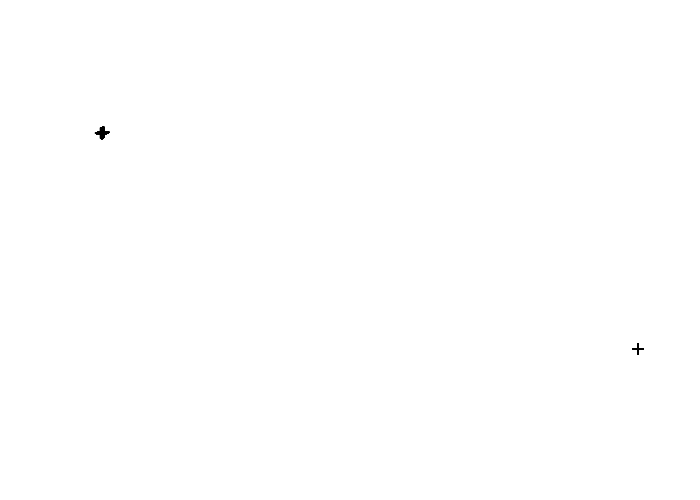Raw Denver Crime Points With Incorrectly Geocoded Points

The plot shows most of the points clustered in a small area at the top of the chart with an aberrant point at the bottom left, probably at (0,0). This indicates the presence of incorrectly geocoded points that must be removed.

Denver also makes available a shapefile of neighborhoods that can be used both for aggregation, and to remove the incorrectly geocoded points.

```neighborhoods = readOGR(dsn='.', layer='statistical_neighborhoods', stringsAsFactors = F)
neighborhoods = spTransform(neighborhoods, wgs84)
plot(neighborhoods, col=terrain.colors(nrow(neighborhoods)))
```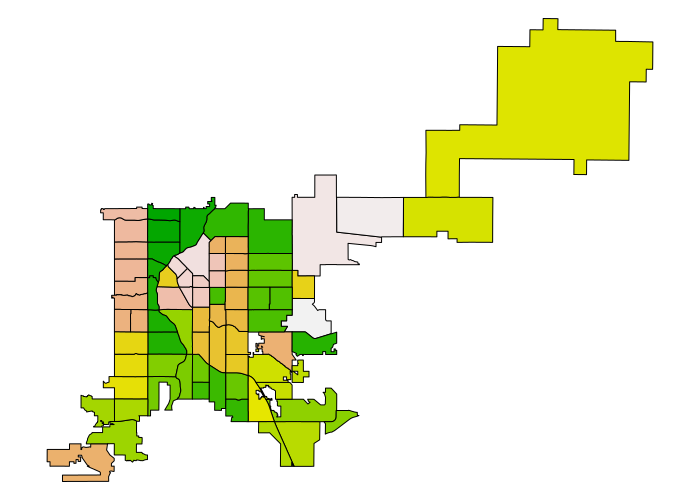Denver Neighborhoods

The over() overlay function can be used to merge the crime points with the neighborhood names, and filter points that do not fall in neighborhoods

```neighborhoods = spTransform(neighborhoods, wgs84)
crime\$NBHD_NAME = over(crime, neighborhoods)\$NBHD_NAME
crime = crime[!is.na(crime\$NBHD_NAME),]

plot(crime, col='#00000010')
plot(neighborhoods, border='gray', add=T)
```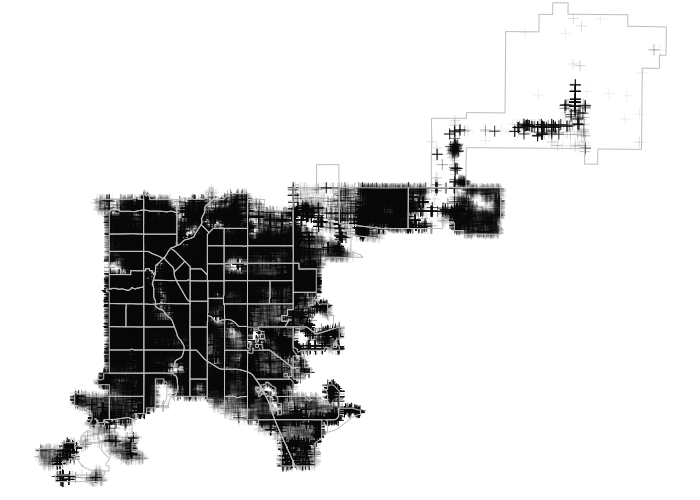Crime Points Constrained to Neighborhoods

Denver is also unusual in that it makes American Community Survey data for census tracts available as a downloadable shapefile. ACS shapefile data can also be created by joining US Census Bureau cartographic boundary shapefiles with a much wider collection of data available through American FactFinder, although the download and join process is somewhat tedious.

```tracts = readOGR(dsn='.', layer='american_community_survey_tracts_2009_2013')
tracts = spTransform(tracts, wgs84)

library(lattice)
trellis.par.set(axis.line=list(col=NA))
spplot(tracts, zcol='MED_HH_INC', col='transparent')
```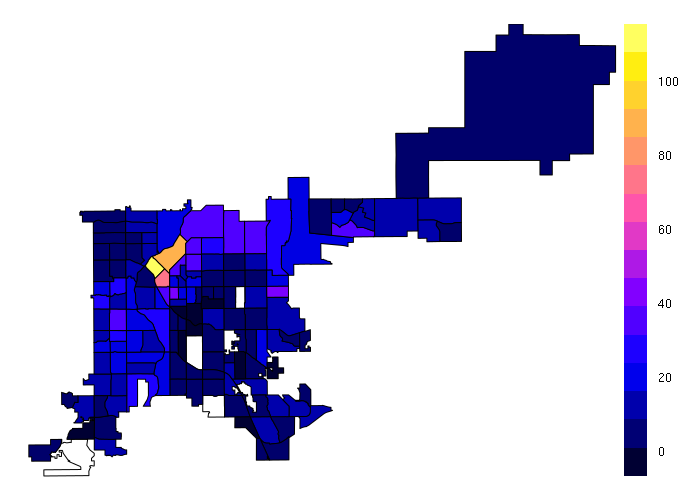2009-2013 ACS Median Household Income by Census Tract in Denver, CO

## Planar Coordinates

Because point analysis often involves distances in physical length units (like feet) rather than degrees (which vary in ground distance depending on where you are on the planet), it is helpful to use a projection like the US State Plane Coordinate System, which treats an area as a flat surface (plane) and then uses coordinates across that surface expressed in feet or meters. The State Plane Coordinate System is commonly used for geospatial data by local governments that need to be able to measure accurate distances on the ground.

You can find the appropriate four-digit State Plane Coordinate System FIPS code for your area of analysis using this map. For example, the FIPS code for the northern half of Washington state is 4601.

You can then get the proj4 string by searching with the FIPS code on SpatialReference.org. Choose the appropriate HARN (High Accuracy Reference Network) projection using the NAD 1983 datum (North American Datum).

```# Colorado Central State Plane 0502
# http://spatialreference.org/ref/esri/102254/
colorado_central = CRS('+proj=lcc +lat_1=38.45 +lat_2=39.75 +lat_0=37.83333333333334
+lon_0=-105.5 +x_0=914401.8289 +y_0=304800.6096 +ellps=GRS80 +units=m +no_defs')

crime = spTransform(crime, colorado_central)
tracts = spTransform(tracts, colorado_central)
neighborhoods = spTransform(neighborhoods, colorado_central)
```

## Selecting Subsets of Data

Many crime data files cover multiple years. Since this can make the data sets large and unweildy, you may want to use selection to limit the data to a specific date range. The exact column names and date formats will depend on your data.

```> nrow(crime)
 420960

> range(crime\$FIRST_OCCURRENCE_DATE)
 "2012-01-02 00:00:00" "2017-04-25 02:30:00"

> crime2015 = crime[(crime\$FIRST_OCCURRENCE_DATE >= '2015') & (crime\$FIRST_OCCURRENCE_DATE < '2016'),]

> range(crime2015\$FIRST_OCCURRENCE_DATE)
 "2015-01-01 00:00:00" "2015-12-31 23:50:00"
```

If your crime data contains one or more columns that looks like a categories, table() can be used to list the different categories and number of entries for each category. These values can be used to subset for analysis of specific crimes.

Subsetting by crime is especially useful in this case since traffic accidents are a dominant part of the Denver data set and make it difficult to see hotspots of other, malicious types of crime.

```> table(crime\$OFFENSE_CATEGORY_ID)

aggravated-assault             all-other-crimes
2006                        15658
arson                   auto-theft
108                         4473
burglary                 drug-alcohol
4857                         6160
larceny                       murder
8849                           57
other-crimes-against-persons              public-disorder
4392                         9753
robbery     theft-from-motor-vehicle
1209                         6226
traffic-accident           white-collar-crime
23229                         1300

> assault2015 = crime2015[crime2015\$OFFENSE_CATEGORY_ID == 'aggravated-assault',]
> plot(assault2015, col="#00000040")
> plot(neighborhoods, border='gray', add=T)
```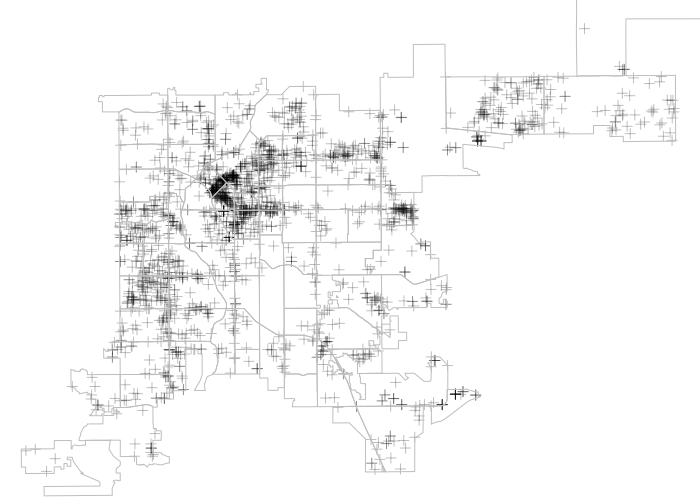2015 Assaults in Denver, CO

## Aggregating by Area

A common and quick way of analyzing crime patterns is by aggregating counts of crimes within areas like census tracts:

```tracts\$ASSAULT2015 = over(tracts, assault2015, fn = length)\$INCIDENT_ID
spplot(tracts, zcol='ASSAULT2015')
```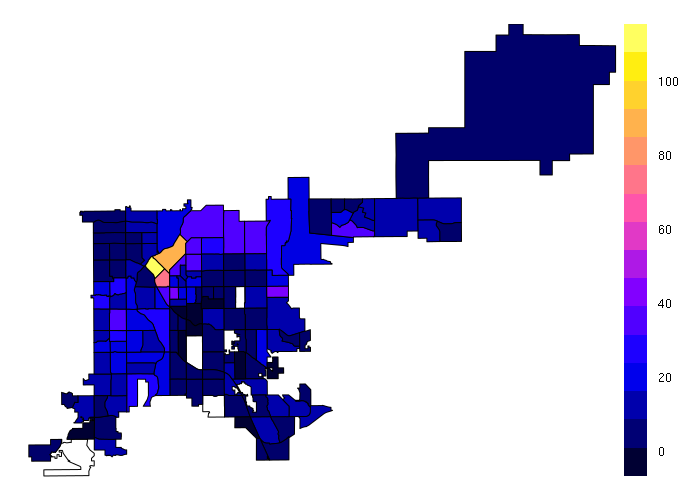2015 Assaults by Census Tract in Denver, CO

While absolute crime numbers can be useful for identifying high crime areas, per capita crime is a more useful statistic that compensates (normalizes) for expected higher levels of crime where there are more people. However, this also tends to overstate crime in areas where there are few residents and large numbers of tourists, such as in Downtown Denver and near the airport.

```tracts\$ASSAULTDENSITY = tracts\$ASSAULT2015 / tracts\$TTL_POPULA
spplot(tracts, zcol='ASSAULTDENSITY')
```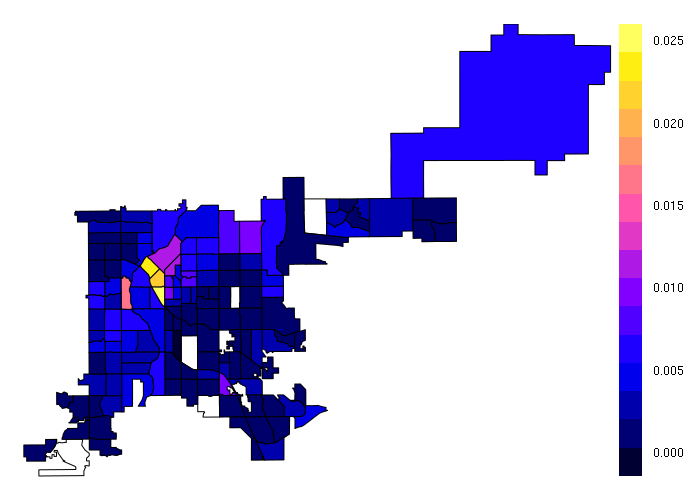2015 Per Capita Assaults by Census Tract Population in Denver, CO

## X-Y Scatter Charts and Histograms

Aggregation by areas like census tracts also allows us to perform analysis of correlation with underlying community characteristics. For example, levels of crime are loosely correlated with poverty, as measured with median household income. A log() transform is used to deal with the skewed distribution of assaults.

```> plot(tracts\$MED_HH_INC, log(tracts\$ASSAULTDENSITY))
> model = lm(log(ASSAULTDENSITY) ~ MED_HH_INC, data=tracts[is.finite(tracts\$ASSAULTDENSITY),])
> abline(model, col='red')
> summary(model)

Call:
lm(formula = log(ASSAULTDENSITY) ~ MED_HH_INC, data = tracts[is.finite(tracts\$ASSAULTDENSITY),])

Residuals:
Min       1Q   Median       3Q      Max
-2.18784 -0.54573 -0.00918  0.52596  2.78741

Coefficients:
Estimate Std. Error t value Pr(>|t|)
(Intercept) -5.033e+00  1.801e-01 -27.944  < 2e-16 ***
MED_HH_INC  -1.948e-05  3.050e-06  -6.387 2.45e-09 ***
---
Signif. codes:  0 ‘***’ 0.001 ‘**’ 0.01 ‘*’ 0.05 ‘.’ 0.1 ‘ ’ 1

Residual standard error: 0.8562 on 137 degrees of freedom
Multiple R-squared:  0.2294,    Adjusted R-squared:  0.2238
F-statistic: 40.79 on 1 and 137 DF,  p-value: 2.45e-09
```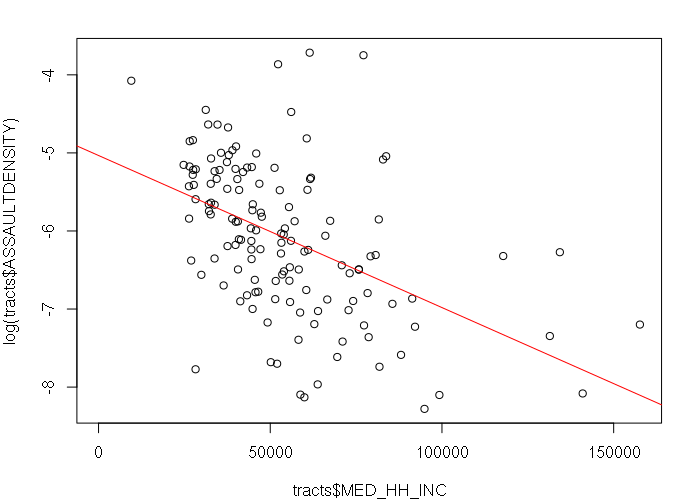2015 Per Capita Assaults vs Median Household Income by Census Tract in Denver, CO

The weakness of that correlation can be visualized by reversing the join and looking at the distribution of median household income where crimes occur in contrast to the distribution of median household income across census tracts.

The histograms look remarkably similar, although the distribution of assaults in areas where the median household income is \$60K or above is noticeably lower than the distribution of income by tract.

```assault2015\$MED_HH_INC = over(assault2015, tracts[,'MED_HH_INC'])\$MED_HH_INC

par(mfrow=c(2,1))
hist(tracts\$MED_HH_INC, breaks = seq(0, 200000, 10000))
hist(assault2015\$MED_HH_INC, breaks = seq(0, 200000, 10000))
```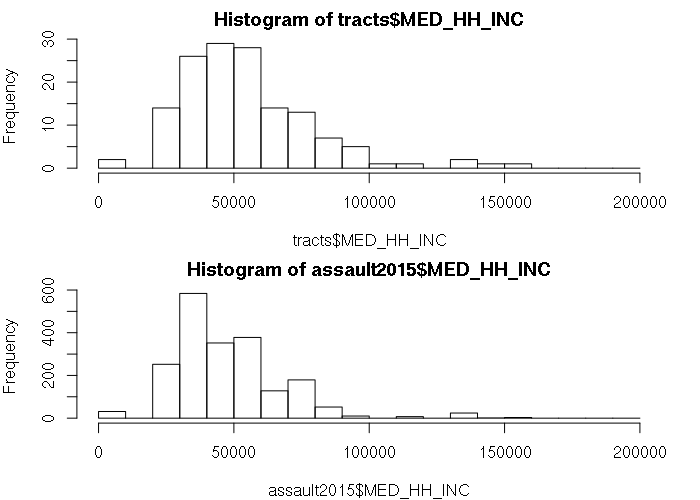2015 Crime vs Tract Median Household Income in Denver, CO

## The Modifiable Areal Unit Problem

A major issue with any kind of aggregation by area is that the results of your analysis can vary widely depending on where you draw the boundaries. This is the Modifiable Areal Unit Problem (MAUP), and can be illustrated by the different patterns displayed when crimes are aggregated by neighborhood rather than by tract. Compensating for the MAUP requires use of techniques like the ones described below.

```neighborhoods\$ASSAULT2015 = over(neighborhoods, assault2015, fn = length)\$INCIDENT_ID
spplot(neighborhoods, zcol='ASSAULT2015')
```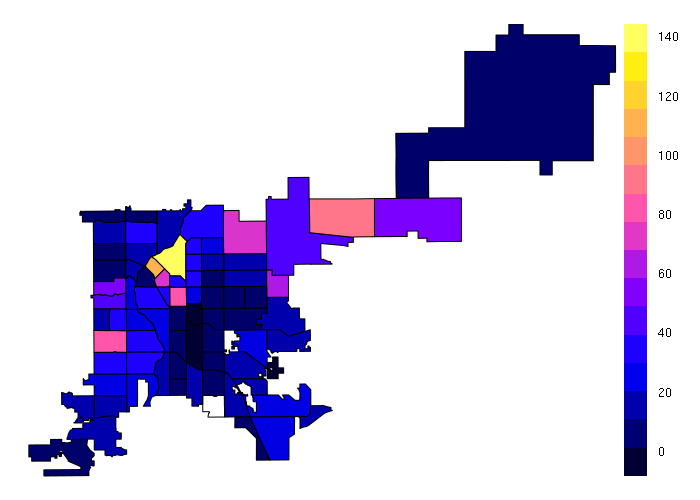2015 Assaults by Neighborhood in Denver, CO

## Heat Maps (Kernel Density Estimation)

One technique that avoids the MAUP is the statistical technique kernel density estimation (KDE). When used with spatial data, KDE is used to create heat maps.

KDE involves systematically running a small kernel matrix over the area being analyzed to visually spread the effects of crime points over adjacent space. On a point map, locations where large numbers of crimes occur close to each other would not be clear, but using a kernel to spread that affect and color it more intensely makes hot spots stand out.

To create a heat map, you start by converting the points to a raster, or regular grid of areas or pixels, using the rasterize() function from the raster library. In this case, since our coordinate system is in feet, a pixel size of 100 means each grid cell is 100 x 100 feet. This choice of size is arbitrary, but should not be too large to avoid making the pixels into areas large enough to run into the MAUP.

Because of this small pixel size, individual crime counts in each pixel are not visually obvious when that raster is visualized.

```library(raster)

pixelsize = 100
box = round(extent(neighborhoods) / pixelsize) * pixelsize
template = raster(box, crs = colorado_central,
nrows = (box@ymax - box@ymin) / pixelsize,
ncols = (box@xmax - box@xmin) / pixelsize)

assault2015\$PRESENT = 1
raster2015 = rasterize(assault2015, template, field = 'PRESENT', fun = sum)
plot(raster2015, xlim=c(950000, 975000), ylim=c(505000, 525000))
plot(neighborhoods, border='#00000040', add=T)
```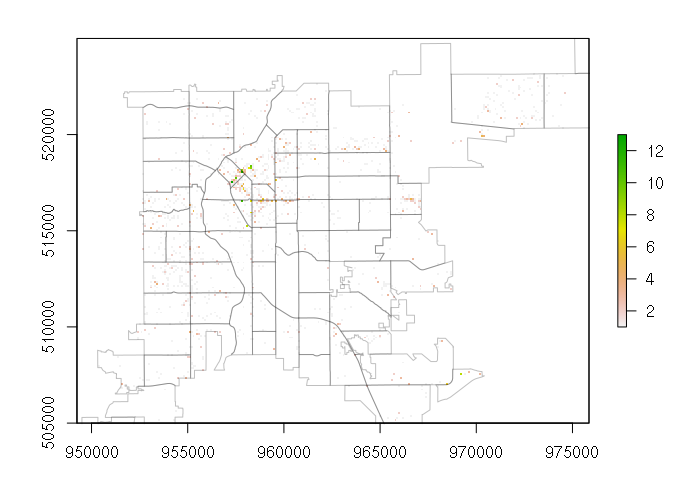Raster of 2015 Assaults in Denver, CO

Next, the focal() function is used to run a Gaussian smoothing kernel created with the focalWeight() function over the raster of crime counts to create a new heat map raster. The heat map clearly shows hot spots of assault in a variety of areas, notably the Downtown area that is home to both a large number of entertainment venues and a significant homeless population.

Because the counts of crime are smoothed over adjacent areas, the intensity of areas relative to other areas on the map is more significant than the absolute numeric values in specific pixels.

```kernel = focalWeight(raster2015, d = 264, type = 'Gauss')
heat2015 = focal(raster2015, kernel, fun = sum, na.rm=T)
plot(heat2015, xlim=c(950000, 975000), ylim=c(505000, 525000))
plot(neighborhoods, border='#00000040', add=T)
```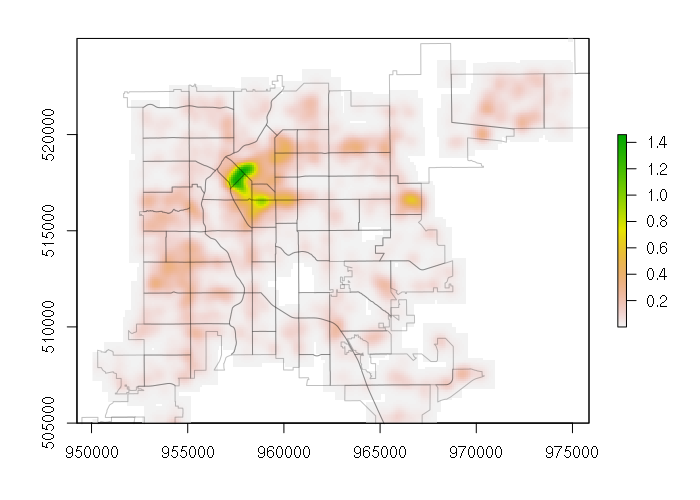Heat Map of 2015 Assaults in Denver, CO

## Contour Lines

In a manner similar to the use of contour lines on an topographic elevation map, contour lines can be created from heat maps to circle hot spots where the level of crime exceeds a certain threshold.

The choice of threshold is made by trial-and-error. Since the absolute value of individual pixel numbers is largely meaningless, there is no absolutely right threshold number, and the choice of an appropriate number requires some knowledge of specific neighborhood conditions to know whether it is too low (including areas that are not really hot spots) or too high (missing important areas of crime concentration). This is a problem similar to the MAUP in that arbitrary or historic choices can significantly alter the results of your analysis.

This code uses the rasterToPolygons() function to create polygons for each pixel where the value exceeds the threshold. The gUnaryUnion() function from the rgeos library is then used to dissolve the lines between adjacent pixel polygons and form outlines. The gBuffer() function is then used to expand and smooth the outlines so they are easier to read.

```library(rgeos)

threshold = 0.15
polygons = rasterToPolygons(x = heat2015, n=16, fun = function(x) { x >= threshold })
contours2015 = gBuffer(gUnaryUnion(polygons), width=100)

plot(heat2015, xlim=c(950000, 975000), ylim=c(505000, 525000))
plot(contours2015, border='blue', add=T)
```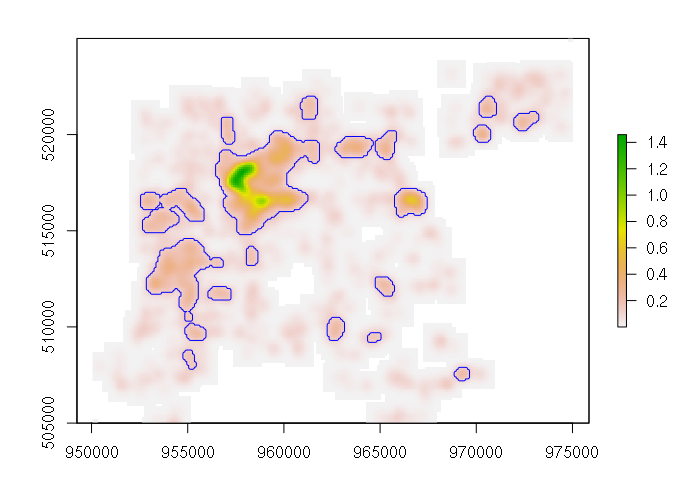Contour Lines for 2015 Assaults in Denver, CO

Contour lines made for different years can also be overlaid to highlight changes in crime hot spots. The blue contours are 2014 hot spots and the red contours are 2015 hot spots. Isolated blue contours are declining areas, isolated red contours are emerging areas, and overlapping red/blue areas are persistent areas of crime.

```crime2014 = crime[(crime\$FIRST_OCCURRENCE_DATE >= '2014') & (crime\$FIRST_OCCURRENCE_DATE < '2015'),]
assault2014 = crime2014[crime2014\$OFFENSE_CATEGORY_ID == 'aggravated-assault',]

assault2014\$PRESENT = 1
raster2014 = rasterize(assault2014, template, field = 'PRESENT', fun = sum)
kernel = focalWeight(raster2014, d = 264, type = 'Gauss')
heat2014 = focal(raster2014, kernel, fun = sum, na.rm=T)

threshold = 0.15
polygons = rasterToPolygons(x = heat2014, n=16, fun = function(x) { x >= threshold })
contours2014 = gBuffer(gUnaryUnion(polygons), width=100)

plot(heat2015, xlim=c(950000, 975000), ylim=c(505000, 525000))
plot(contours2014, border='blue', add=T)
plot(contours2015, border='red', add=T)
```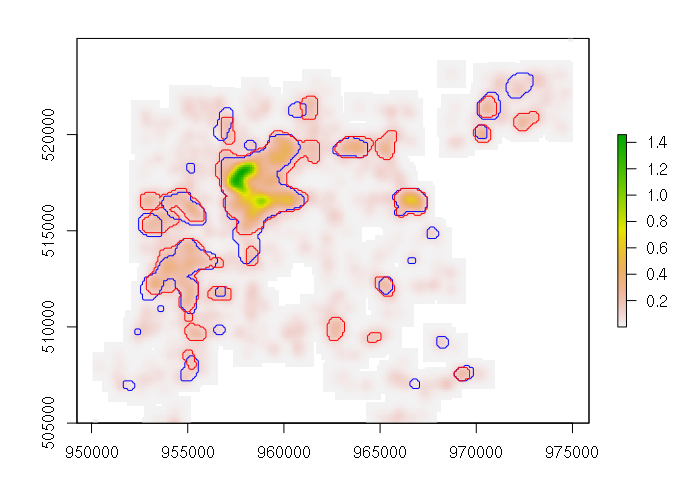Contour Lines Comparing 2014 and 2015 Assaults in Denver, CO

## Getis-Ord GI*

One major issue with kernel density estimation is that the choice of a threshold for what is and is not a hot spot is arbitrary, making use of KDE imprecise and subject to misinterpretation.

One commonly used technique to work around that issues was developed by Arthur Getis and JK Ord in the early 1990s. When you develop an algorithm, you get to name it after yourself, so this is called the Getis-Ord GI* statistic.

This statistic is based on the observation that the distribution of differences in crime intensity between neighborhing areas across a map will follow a normal curve. Therefore, the amount of difference between an area and its neighbors can then be converted to a z-score reflecting the number of standard deviations (σ) that the crime level in an area differs from neighborhood normal. Areas with high z-scores (indicating a crime significantly above the mean) are identified as hot spots, while areas with low z-scores are identified as cool spots.

```# Create a regular grid of 1/8-mile-square crime areas via a raster

pixelsize = 660
box = round(extent(neighborhoods) / pixelsize) * pixelsize
template = raster(box, crs = colorado_central,
nrows = (box@ymax - box@ymin) / pixelsize,
ncols = (box@xmax - box@xmin) / pixelsize)

assault2015\$PRESENT = 1
getisraster = rasterize(assault2015, template, field = 'PRESENT', fun = sum)
getisgrid = rasterToPolygons(getisraster)

# Create the list of neighbors

library(spdep)
neighbors = poly2nb(getisgrid)
weighted_neighbors = nb2listw(neighbors, zero.policy=T)

# Perform the local G analysis (Getis-Ord GI*)

getisgrid\$HOTSPOT = as.vector(localG(getisgrid\$layer, weighted_neighbors))

# Color the grid cells based on the z-score

breaks = c(-20, -1.96, -1, 1, 1.96, 20)
palette=c("#0000FF80", "#8080FF80", "#FFFFFF80", "#FF808080", "#FF000080")
col = palette[cut(getisgrid\$HOTSPOT, breaks)]

# Plot

plot(getisgrid, col=col, border=NA, xlim=c(950000, 975000), ylim=c(505000, 525000))
plot(neighborhoods, border="gray", add=T)
```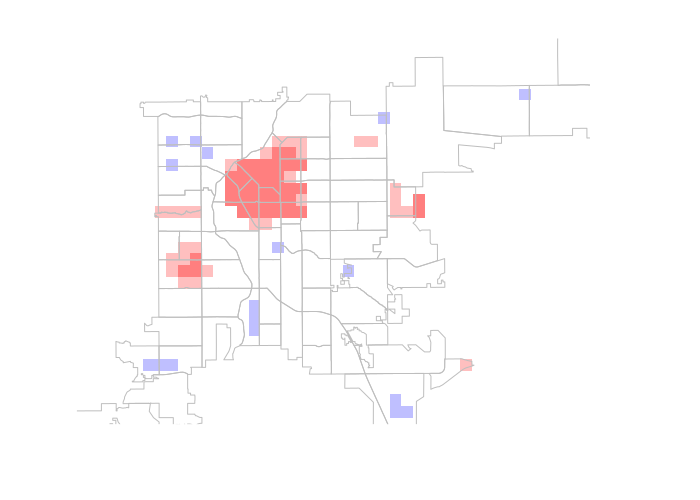Getis-Ord GI* Hot Spot Analysis of 2015 Assaults in Denver, CO

## Base Map

Crime mapping has practical application as a tool for knowing where resources and interventions should be directed. Crime maps need to have geographic context so the results can be associated with specific, knowable areas of the community. Therefore, crime maps need base maps that help readers know how the results of analysis relate to specific locations.

It is possible to assemble customized base maps with application-specific details, but such construction is tedious in R and may be best performed with interactive software like ArcMap, QGIS, and/or Adobe Illustrator.

However, the use of OpenStreetMap as a base map is an easy, albeit imperfect, way to generate quick base maps.

The following examples presume creation of the raster and spatial objects as described in the sections above, and only address plotting over OSM.

### The OSM Base Map

```library(OpenStreetMap)
basemap = openmap(upperLeft = c(39.82, -105.1), lowerRight = c(39.6, -104.8), type="osm")
basemap = openproj(basemap, colorado_central)
plot(basemap)
```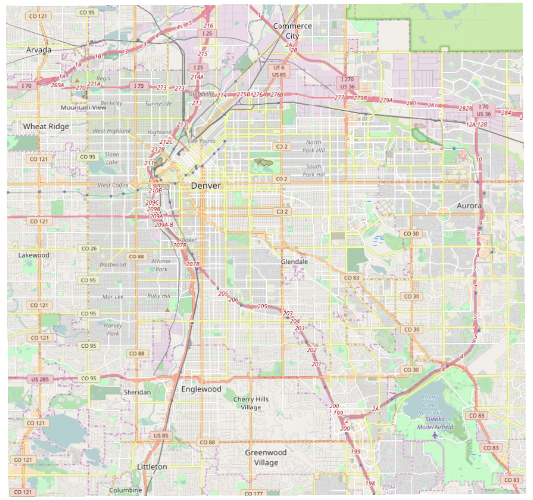OpenStreetMap of Denver, CO

### Semitransparent Tract Areas

```plottracts = tracts[!is.infinite(tracts\$ASSAULTDENSITY),]
plottracts\$ASSAULTDENSITY = 1000 * plottracts\$ASSAULTDENSITY

palette = colorRampPalette(c("#0000FF80", "#FF000080"), alpha=T)(4)
breaks = quantile(plottracts\$ASSAULTDENSITY, na.rm=T)
col = palette[cut(plottracts\$ASSAULTDENSITY, breaks)]

plot(basemap)
plot(plottracts, col=col, border='gray', add=T)

labels = sapply(1:4, function(z) paste(round(breaks[z], 2), '-', round(breaks[z+1], 2)))
legend(x = 'bottomright', inset = 0.05, bg = 'white', legend = labels, col = palette, pch = 15)
```2015 Assaults Per 1,000 Residents by Census Tract in Denver, CO

### Heat Map

```image = brick(heat2015, nl=3, values = F)
ramp = colorRamp(c("blue", "red"))
values(image) = ramp(values(heat2015) / max(values(heat2015), na.rm=T))

plot(basemap)
plotRGB(image, alpha = 192, bgalpha=0, add=T)
```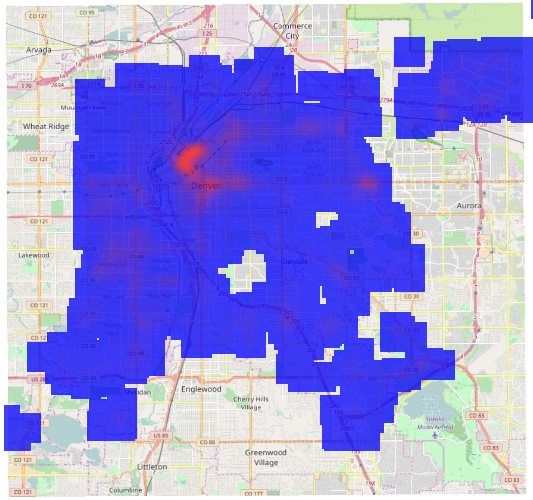Heat Map of Assaults in 2015 in Denver, CO

### Contour Outlines

```plot(basemap)
plot(contours2014, border='blue', lwd=3, add=T)
plot(contours2015, border='red', lwd=3, add=T)
```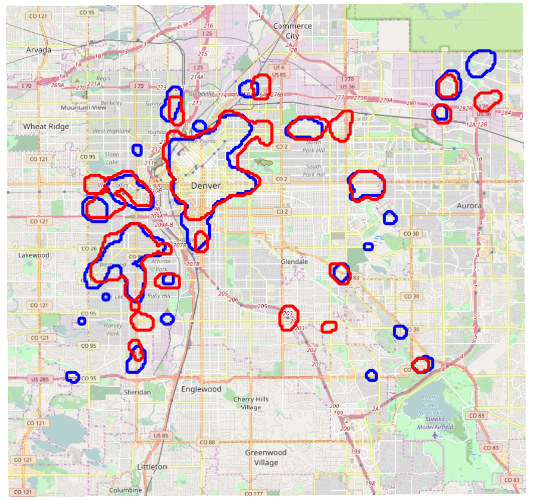2014 vs 2015 Assault Hot Spot Contours in Denver, CO

### Getis-Ord Grid

```breaks = c(-20, -1.96, -1, 1, 1.96, 20)
palette=c("#0000FFC0", "#8080FFC0", "#FFFFFF80", "#FF8080C0", "#FF0000C0")
col = palette[cut(getisgrid\$HOTSPOT, breaks)]

plot(basemap)
plot(getisgrid, col=col, border=NA, add=T)

legend(x='bottomright', inset=0.05, bg = 'white', col=palette, pch=15,
legend = c('Cold Spot >99% Confidence', 'Cold Spot 68-95% Confidence',
'Not Significant', 'Hot Spot 68 - 95% Confidence',
'Hot Spot >95% Confidence'))
```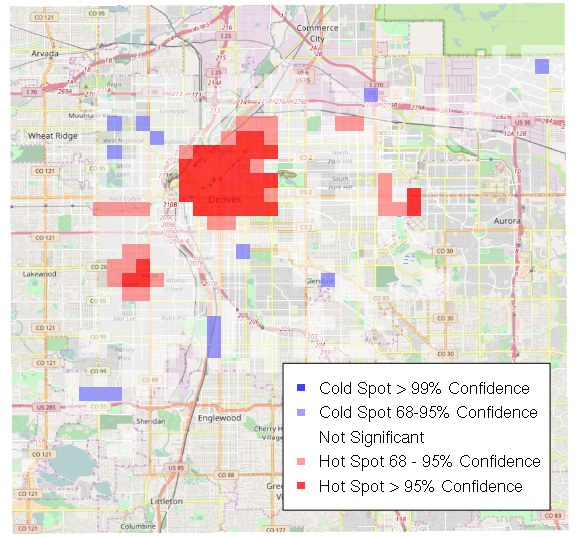Getis-Ord GI* Hot Spot Analysis of 2015 Assaults in Denver, CO

### Downtown Closeup

```downtown = openmap(upperLeft = c(39.765, -105.005), lowerRight = c(39.737, -104.959), type="osm")
downtown = openproj(downtown, colorado_central)
plot(downtown)
plot(assault2015, col='#A00000A0', pch=19, add=T)
```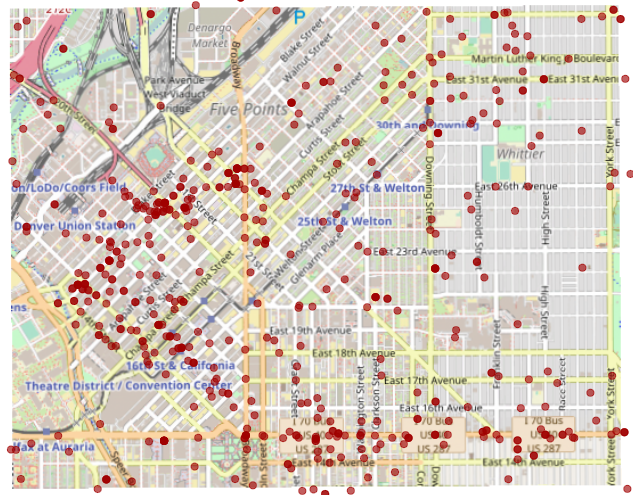2015 Assaults in Downtown Denver, CO